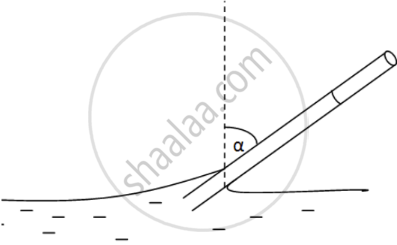Advertisement Remove all ads

# Water Rises in a Vertical Capillary Tube up to a Length of 10 Cm. If the Tube is Inclined at 45°, the Length of Water Risen in the Tube Will Be - Physics

MCQ

Water rises in a vertical capillary tube up to a length of 10 cm. If the tube is inclined at 45°, the length of water risen in the tube will be

#### Options

• 10 cm

• $10\sqrt{2}$ cm

• $10/\sqrt{2}$ cm

•  none of these

Advertisement Remove all ads

#### Solution$\text{ Given }:$

$\text{ l = 10 cm }$

$\alpha = {45}^0$

$\text{ Rise in water level after the tube is tilted = h}$

$\Rightarrow \text{ l = h} \cos {45}^0$

$\Rightarrow h = \frac{l}{\cos {45}^0} = \frac{10}{\left( \frac{1}{\sqrt{2}} \right)} = 10\sqrt{2}\text{ cm }$

Is there an error in this question or solution?
Advertisement Remove all ads

#### APPEARS IN

HC Verma Class 11, 12 Concepts of Physics 1
Chapter 14 Some Mechanical Properties of Matter
MCQ | Q 21 | Page 299
Advertisement Remove all ads

#### Video TutorialsVIEW ALL 

Advertisement Remove all ads
Share
Notifications

View all notifications

Forgot password?